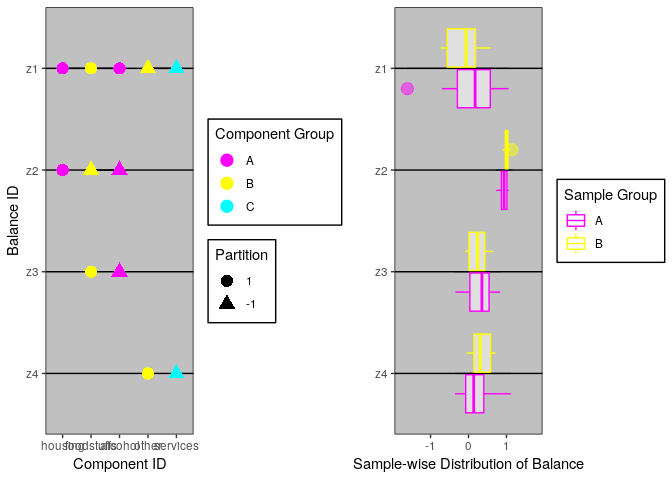# Visualize Balances of Compositional Data

## Quick start

Welcome to the balance GitHub page!

Balances have become a cornerstone of compositional data analysis. However, conceptualizing balances is difficult, especially for high-dimensional data. Most often, investigators visualize balances with “balance dendrograms”. However, this visualization tool does not scale well for large data. This package provides an alternative scheme for visualizing balances. This package also provides a method for principal balance analysis.

library(devtools)
devtools::install_github("tpq/balance")
library(balance)
?balance

## Generating balance figures

We will demonstrate this package using an example from the robCompositions package. The “expenditures” matrix contains 20 compositions (row), each measuring 5 components (columns). As compositional data, the abundances are irrelevant and each composition sums to unity. The “y1” matrix is a serial binary partition (SBP) matrix that describes how to partition the 5 components into 4 balances.

data(expenditures, package = "robCompositions")
y1 <- data.frame(c(1, 1, 1, -1, -1), c(1, -1, -1, 0, 0),
c(0, +1, -1, 0, 0), c(0, 0, 0, +1, -1))
colnames(y1) <- paste0("z", 1:4)

With the data loaded, we can calculate and visualize the balances.

res <- balance.plot(expenditures, y1, size.text = 8)
#> Registered S3 methods overwritten by 'ggplot2':
#>   method         from
#>   [.quosures     rlang
#>   c.quosures     rlang
#>   print.quosures rlang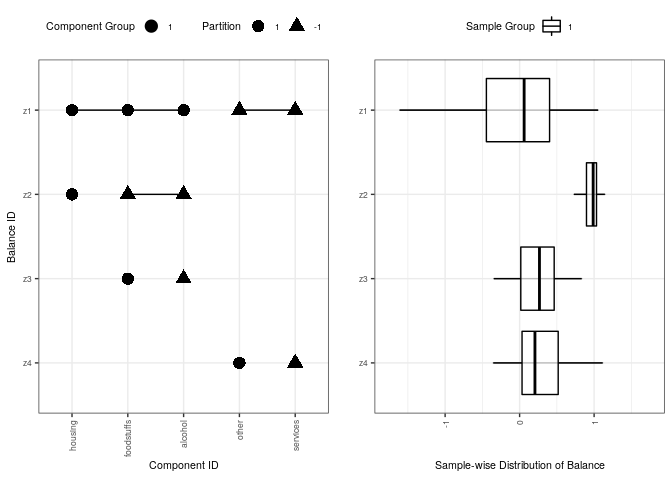Optionally, users can color components (in left figure) or samples (in right figure) based on a user-defined grouping. To do this, users must provide a vector of group labels for each component via the d.groupargument (or for each sample via the n.group argument). Though, due to limitations imposed by the palette, there is a maximum of eight groupings allowed per vector.

Here, we color components by a user-defined grouping.

res <- balance.plot(expenditures, y1, d.group = c("A", "B", "A", "B", "C"), size.text = 8)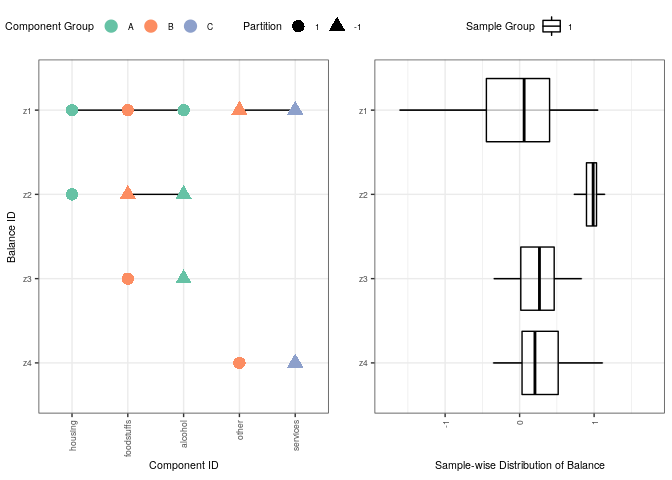Here, we color samples by a user-defined grouping.

res <- balance.plot(expenditures, y1, n.group = c(rep("A", 10), rep("B", 10)), size.text = 8)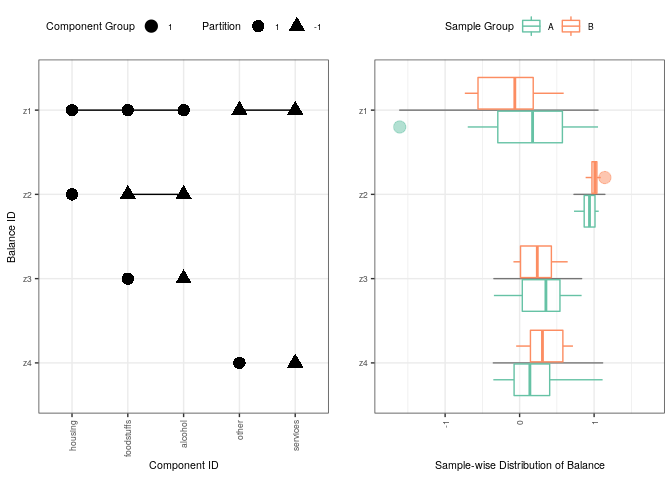Here, we do both.

res <- balance.plot(expenditures, y1,
d.group = c("A", "B", "A", "B", "C"),
n.group = c(rep("A", 10), rep("B", 10)),
size.text = 8)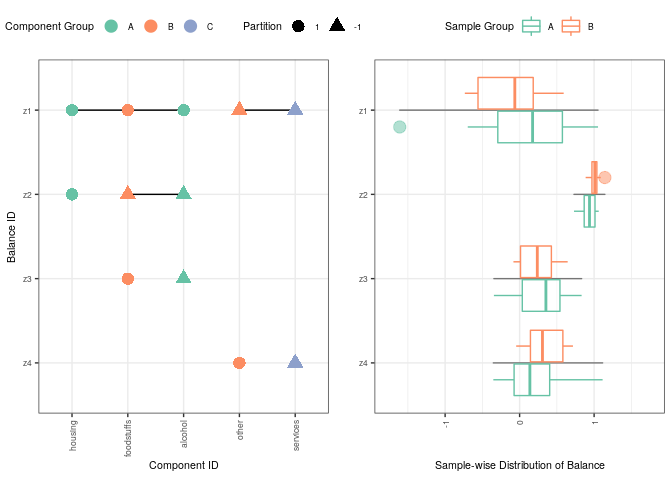## Modifying balance figures

The balance.plot function returns a list containing the “partition” ggplot object, the “distribution” ggplot object, and the per-sample balances.

library(ggthemes)
custom1 <- res[] + theme_excel() + scale_colour_excel()
balance.combine(custom1, custom2)